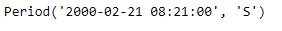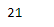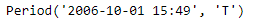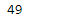Python | Pandas Period.minute

• Last Updated : 06 Jan, 2019

Python is a great language for doing data analysis, primarily because of the fantastic ecosystem of data-centric python packages. Pandas is one of those packages and makes importing and analyzing data much easier.

Pandas Period.minute attribute return an integer value. The returned value represents the number of minutes in the given Period object.

Syntax : Period.minute

Parameters : None

Return : number of minutes

Example #1: Use Period.minute attribute to find the minute value present in the given Period object.

 # importing pandas as pdimport pandas as pd  # Create the Period objectprd = pd.Period(freq ='S', year = 2000, month = 2, day = 21, hour = 8, minute = 21)  # Print the Period objectprint(prd)

Output :Now we will use the Period.minute attribute to find out the minute value present in prd object.

 # find minute valueprd.minute

Output :As we can see in the output, the Period.minute attribute has returned 21 indicating the minute value present in the given period object is 21.

Example #2: Use Period.minute attribute to find the minute value present in the given Period object.

 # importing pandas as pdimport pandas as pd  # Create the Period objectprd = pd.Period(freq ='T', year = 2006, month = 10,                            hour = 15, minute = 49)  # Print the Period objectprint(prd)

Output :Now we will use the Period.minute attribute to find out the minute value present in prd object.

 # find minute valueprd.minute

Output :As we can see in the output, the Period.minute attribute has returned 49 indicating the minute value present in the given period object is 49.

My Personal Notes arrow_drop_up